Function Repository Resource:

# TetrahedronEdgeAngles

Given edge lengths of a tetrahedron, return the dihedral angles opposite those edges

Contributed by: Wolfram Staff
 ResourceFunction["TetrahedronEdgeAngles"][{a,b,c,d,e,f}] returns the angles opposite edges (a,b,c,d,e,f).

## Examples

### Basic Examples (2)

Here is a rare tetrahedron where all dihedral angles are nice:

 In:=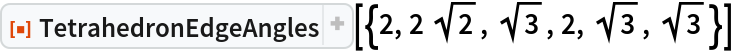Out=A set of vertices for the above tetrahedron:

 In:=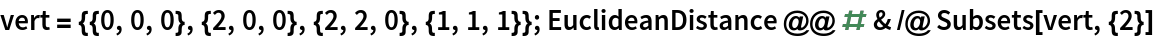Out=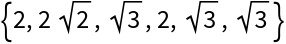Opposing edges are 1–6, 2–5 and 3–4:

 In:=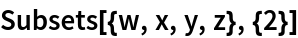Out=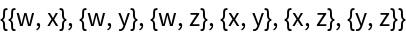Usually the angles are messier:

 In:=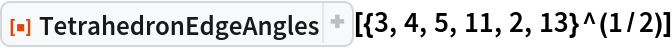Out=### Scope (1)

Another tetrahedron with nicer angles:

 In:=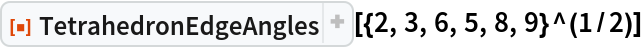Out=## Requirements

Wolfram Language 11.3 (March 2018) or above

## Version History

• 1.0.0 – 11 February 2019